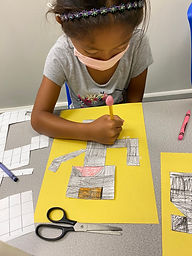## Ms. Brianne

### Target 1​

###### Lesson Type:

New

Number Operation

:

Computation

Divide within 100.

###### 1:

Understand that dividing numbers makes numbers smaller.

###### 2:

Understand that division represents taking a quantity and determining how many equal groups of objects can be made.

###### 3:

Understand there are multiple ways to divide a multi-digit number.

3rd

###### Vocabulary:

Divide, Equal Groups, Skip Counting, Repeated Subtraction, Fact Families

Activities:

• Students learned that division determines how many equal groups there are in a quantity.
• Students were given a quantity of pictures and a division situation. They circled a given number to help them find out how many groups could be made with the quantity.
• Students were introduced to different strategies they could use to divide (equal groups, skip counting, repeated subtraction, fact families). They chose the strategy they wanted to use to solve division problems.### Home Exploration

###### Guiding Questions:## Absent Students:

### Target 2

:

###### 1:

Relate area to arithmetic operations (multiplication and addition).

###### 2:

Recognize area as a measurement of 2D shapes.

3rd

###### Vocabulary:

Area, Length, Width, Multiply, Square Units, Half Unit

Activities:

• Students practiced finding the area of 2D shapes that included half-units.
• Students created their own robots using grid paper. They had to use full and half-unit squares in their robot. Then they found the area of their robot.
• Students used cubes to create different rectangles on an array board. Then they wrote the perimeter and area for their rectangle. They found that they could multiply the length by the width to find the area and included the matching multiplication equation.### Home Exploration

###### Guiding Questions:### Target 3

:

###### Vocabulary:

Activities:### Home Exploration